• 箱形图 python Python-箱形图 (Python - Box Plots) Advertisements 广告 Previous Page 上一页 Next Page 下一页 Boxplots are a measure of how well distributed the data in a data set is. It ...箱形图 python
Python-箱形图 (Python - Box Plots)
Boxplots are a measure of how well distributed the data in a data set is. It divides the data set into three quartiles. This graph represents the minimum, maximum, median, first quartile and third quartile in the data set. It is also useful in comparing the distribution of data across data sets by drawing boxplots for each of them.
箱线图是衡量数据在数据集中的分布程度的一种度量。 它将数据集分为三个四分位数。 该图表示数据集中的最小，最大，中位数，第一四分位数和第三四分位数。 通过为每个数据集绘制箱形图来比较数据在数据集之间的分布，这也很有用。
绘制箱形图 (Drawing a Box Plot)
Boxplot can be drawn calling Series.box.plot() and DataFrame.box.plot(), or DataFrame.boxplot() to visualize the distribution of values within each column.
可以通过调用Series.box.plot()和DataFrame.box.plot()或DataFrame.boxplot()来绘制Boxplot，以可视化每个列中值的分布。
For instance, here is a boxplot representing five trials of 10 observations of a uniform random variable on [0,1).
例如，这是一个箱线图，代表对[0,1)上的一个随机变量的10个观测值的五个试验。

import pandas as pd
import numpy as np
df = pd.DataFrame(np.random.rand(10, 5), columns=['A', 'B', 'C', 'D', 'E'])
df.plot.box(grid='True')


Its output is as follows −
其输出如下-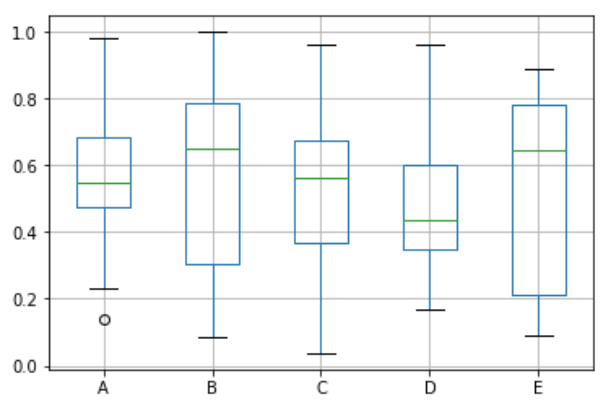翻译自: https://www.tutorialspoint.com/python_data_science/python_box_plots.htm

箱形图 python

展开全文可视化 机器学习 数据挖掘 数据分析
• ## 箱形图（python画箱线图）

万次阅读 多人点赞 2019-04-18 21:34:17
箱形图 价值 局限性 Python画图 箱形图 如下灰色框里的就是箱形图（英文：Box plot）：又称为盒须图、盒式图、盒状图或箱线图，是一种用作显示一组数据分散情况资料的统计图。因型状如箱子而得名。 箱形图最大...
学习笔记
目录
箱形图
价值
局限性
Python画图

箱形图
如下灰色框里的就是箱形图（英文：Box plot）：又称为盒须图、盒式图、盒状图或箱线图，是一种用作显示一组数据分散情况资料的统计图。因型状如箱子而得名。
箱形图最大的优点就是不受异常值的影响，可以以一种相对稳定的方式描述数据的离散分布情况。
五数概括法：即用下面的五个数来概括数据（最小值；第1四分位数(Q1)；中位数(Q2)；第3四分位数(Q3)；最大值），箱形图与之类似。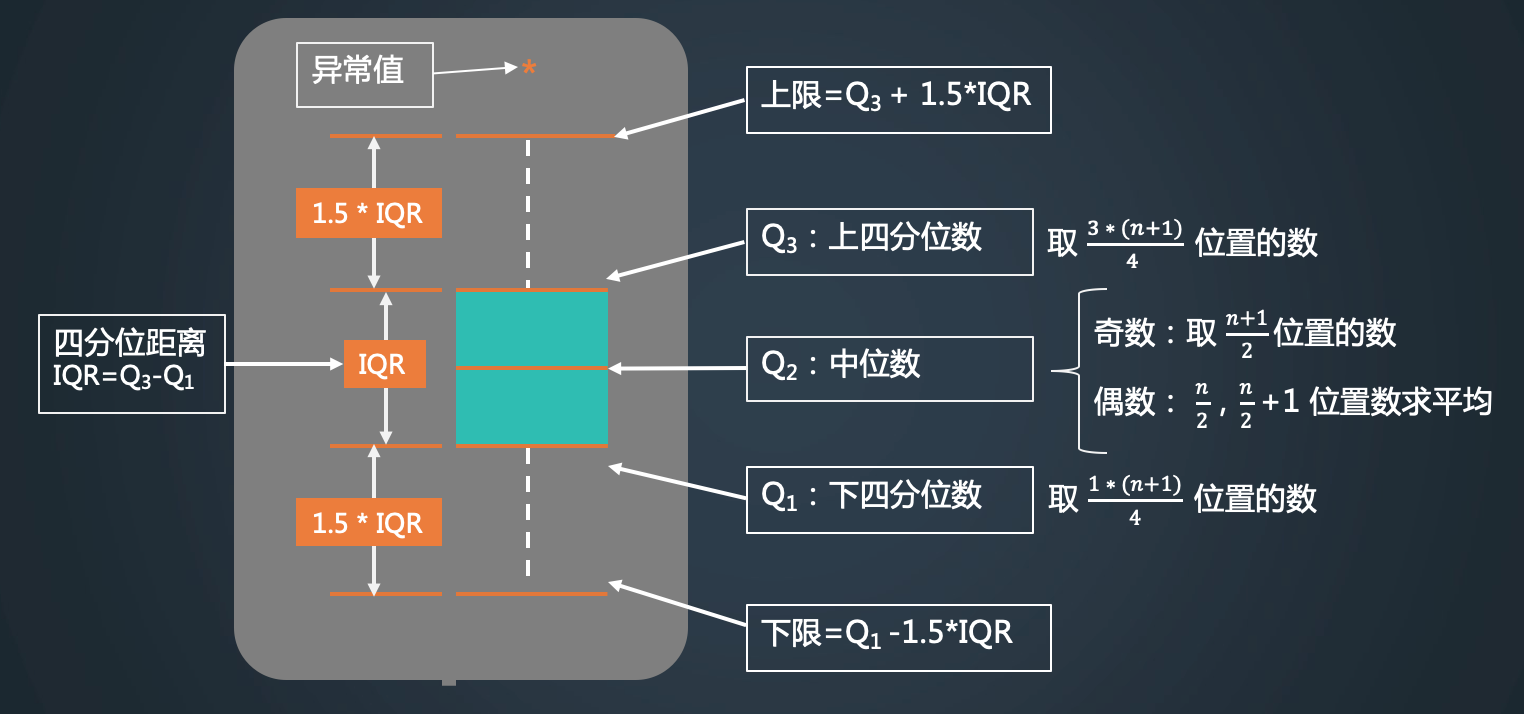举个栗子：num=[1,2,3,4,5,6,7,8]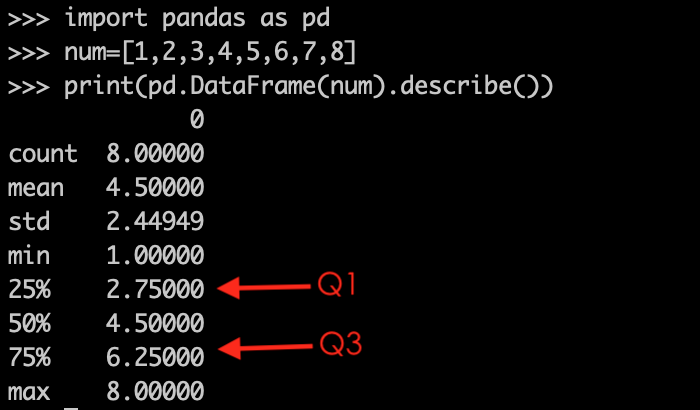中位数Q2=4.5
下四分分位数Q1=2.75：是怎么计算出来的呢？首先序列长度n=8，(1+n)/4=2.25，说明上四分位数在第2.25个位置数，实际上这个数是不存在的，但我们知道这个位置是在第2个数与第3个数之间的。假设从第2个数到第3个数之间是均匀分布的。那么第2.25个数就是第二个数*0.25+第三个数*0.75，即2*0.25+3*0.75=0.5+2.25=2.75。 下四分位数Q3=6.25：这个下四分位数所在位置计算方法同上，只不过是(1+n)/4*3=6.75，这个是个介于第六个位置与第七个位置之间的地方。对应的具体的值是0.75*6+0.25*7=6.25。
四分位距IQR=Q3-Q1，上限=（Q3+1.5IQR，max）取最小，下限=（Q1-1.5IQR ，min)取最大。
异常值：比如num=[1,2,3,4,5,6,7,8,20]，20会被识别为异常值。
价值
序号价值备注1直观明了地识别数据批中的异常值箱线图判断异常值的标准以四分位数和四分位距为基础，四分位数具有一定的耐抗性，多达25%的数据可以变得任意远而不会很大地扰动四分位数，所以异常值不会影响箱形图的数据形状，箱线图识别异常值的结果比较客观。由此可见，箱线图在识别异常值方面有一定的优越性。2利用箱线图判断数据批的偏态和尾重 对于标准正态分布的样本，只有极少值为异常值。异常值越多说明尾部越重，自由度越小（即自由变动的量的个数）； 而偏态表示偏离程度，异常值集中在较小值一侧，则分布呈左偏态；异常值集中在较大值一侧，则分布呈右偏态。3利用箱线图比较几批数据的形状 同一数轴上，几批数据的箱线图并行排列，几批数据的中位数、尾长、异常值、分布区间等形状信息便昭然若揭。如上图，可直观得看出第三季度各分公司的销售额大体都在下降。 import numpy as np
import pandas as pd
import matplotlib.pyplot as plt

data = {
'China': [1000, 1200, 1300, 1400, 1500, 1600, 1700, 1800, 1900, 2500],
'America': [1200, 1300, 1400, 1500, 1600, 1700, 1800, 1900, 2000, 2100],
'Britain': [1000, 1200, 1300, 1400, 1500, 1600, 1700, 1800, 1900, 2000],
"Russia": [800, 1000, 1200, 1300, 1400, 1500, 1600, 1700, 1800, 1900]
}

df = pd.DataFrame(data)

df.plot.box(title="Consumer spending in each country")
plt.grid(linestyle="--", alpha=0.3)
plt.show() 各国的消费（代码来源于https://www.cnblogs.com/wwxbi/p/9032248.html）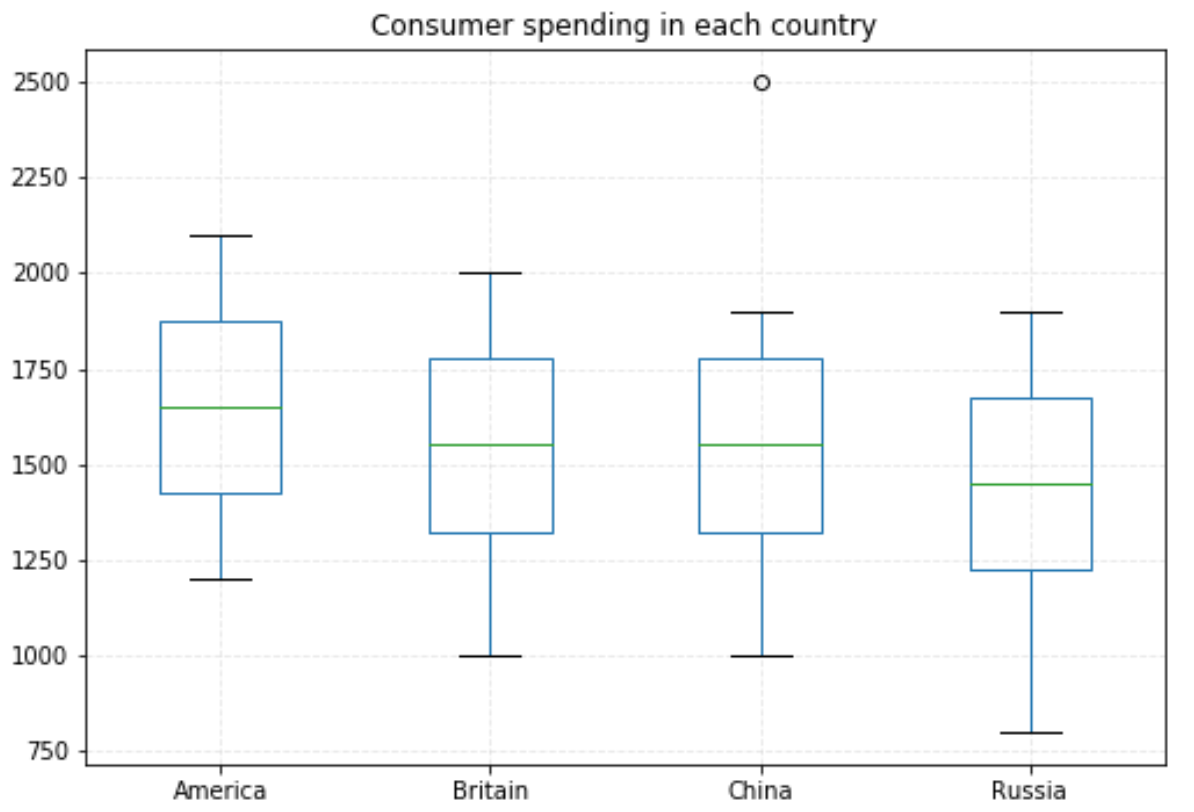局限性
不能精确地衡量数据分布的偏态和尾重程度；对于批量比较大的数据，反映的信息更加模糊以及用中位数代表总体评价水平有一定的局限性
Python画图
在pyton命令行直接运行就OK
import numpy as np
import pandas as pd
import matplotlib.pyplot as plt

data = [1200, 1300, 1400, 1500, 1600, 1700, 1800, 1900, 2000, 2100]

df = pd.DataFrame(data)
df.plot.box(title="hua tu")
plt.grid(linestyle="--", alpha=0.3)
plt.show()

箱形图描述数据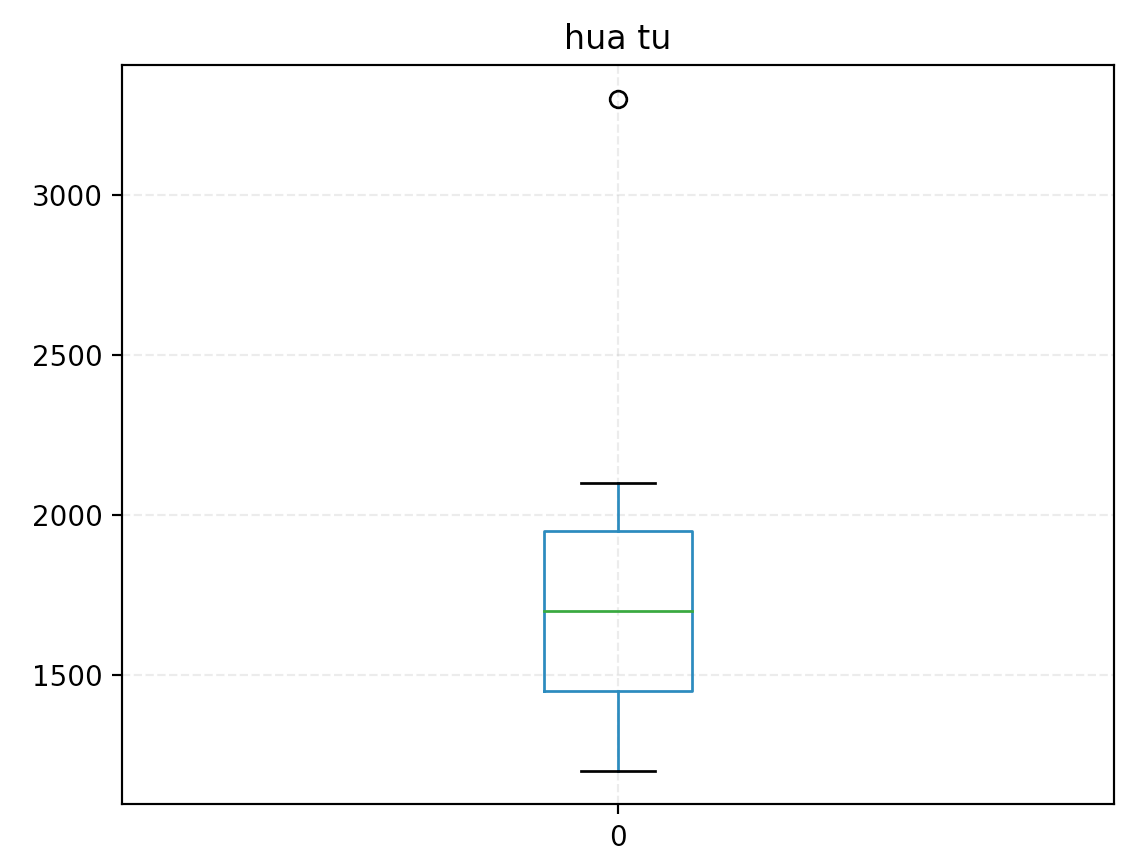五数概括法:描述数据
import numpy as np
import pandas as pd
import matplotlib.pyplot as plt

data = [1200, 1300, 1400, 1500, 1600, 1700, 1800, 1900, 2000, 2100]
df = pd.DataFrame(data)
print(df.describe())
count    10.000000    # 条数
mean   1650.000000    # 均值
std     302.765035    # 标准差
min    1200.000000    # 最小值
25%    1425.000000    # 下四分位
50%    1650.000000    # 中位数
75%    1875.000000    # 上四分位
max    2100.000000    # 最大值

展开全文统计学
• ## Python实现箱形图的绘制

万次阅读 多人点赞 2018-11-07 18:49:02
因为箱形图是基于五数概括法的图形汇总，在介绍箱形图前，先简单介绍一下五数概括法。 五数概括法使用下面五个数来汇总数据： （1）最小值(Q1-1.5IQR) （2）第一四分位数(Q1) （3）中位数(Q2) （4）第三四分...
说明：代码运行环境为 Win10+Python3+jupyter notebook
箱形图简介：
因为箱形图是基于五数概括法的图形汇总，在介绍箱形图前，先简单介绍一下五数概括法。
五数概括法使用下面五个数来汇总数据：
（1）最小值(Q1-1.5IQR)
（2）第一四分位数(Q1)
（3）中位数(Q2)
（4）第三四分位数(Q3)
（5）最大值(Q3+1.5IQR)
而箱形图就是五数概括法的图形表示。如下图所示：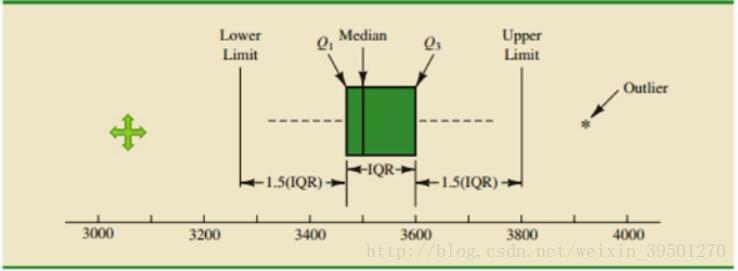（图片来源：https://blog.csdn.net/weixin_39501270/article/details/77369597）
箱形图的应用：
（1）可以作为一种检测异常值的方法；
（2）用于多组数据的图形汇总，便于对各组数据进行直观比较分析。
箱形图的绘制方法主要有：
方法1：利用pandas包中的Series.plot()、DataFrame.plot()或DataFrame.boxplot()方法；
方法2：利用seaborn包中的cataplot()或者boxplot()，其中seaborn.boxplot()是seaborn.cataplot()的参数kind='box'时的一种情况；
方法3：利用matplotlib包中axes对象的boxplot()方法。
导出需要的各种包，并准备好数据：
%matplotlib notebook
import matplotlib.pyplot as plt
import pandas as pd
import numpy as np
import seaborn as

tips['tip_pct'] = tips['tip'] / (tips['total_bill'] - tips['tip'])
tips的表结构为：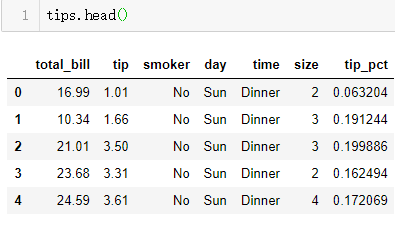方法1具体示例：
Series.plot()示例：
fig,axes = plt.subplots()
tips['tip_pct'][tips.tip_pct < 0.5].plot(kind='box',ax=axes)
axes.set_ylabel('values of tip_pct')
fig.savefig('p1.png')    # 将绘制的图形保存为p1.png
上述代码绘制的图形为：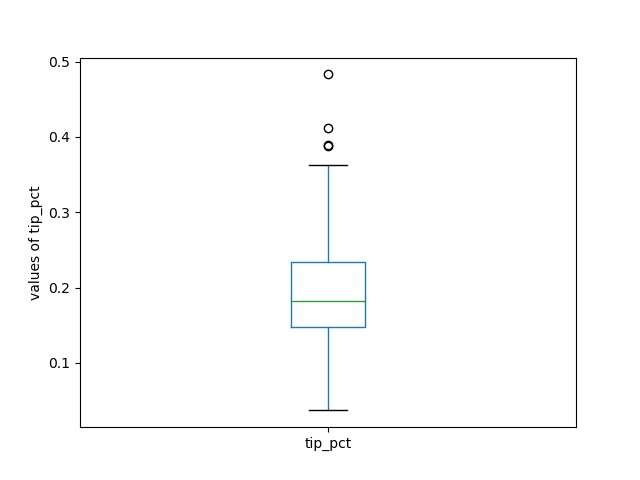Series.plot()的用法具体参考：
https://pandas.pydata.org/pandas-docs/stable/generated/pandas.Series.plot.html#pandas.Series.plot
DataFrame.plot()示例：
fig,axes = plt.subplots(1,4)
color = dict(boxes='DarkGreen', whiskers='DarkOrange',
medians='DarkBlue', caps='Red')
# boxes表示箱体，whisker表示触须线
# medians表示中位数，caps表示最大与最小值界限

tips.plot(kind='box',ax=axes,subplots=True,
title='Different boxplots',color=color,sym='r+')
# sym参数表示异常值标记的方式

axes.set_ylabel('values of total_bill')
axes.set_ylabel('values of tip')
axes.set_ylabel('values of size')
axes.set_ylabel('values of tips_pct')

fig.savefig('p2.png')    # 将绘制的图片保存为p2.png
上述代码绘制的图形为：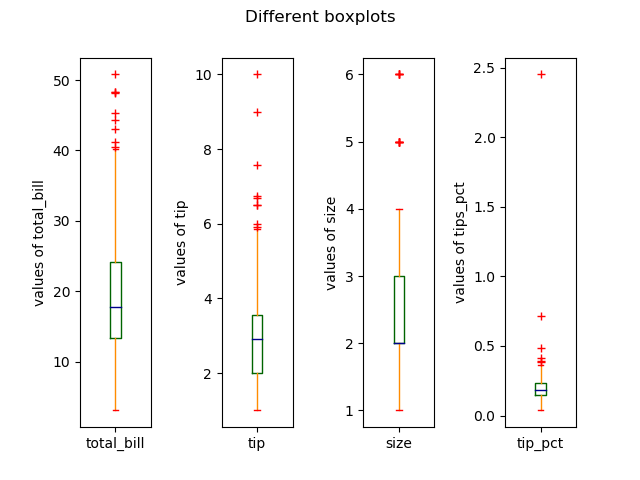DataFrame.plot()的用法具体参考：
https://pandas.pydata.org/pandas-docs/stable/generated/pandas.DataFrame.plot.html
DataFrame.boxplot()示例：
fig,axes = plt.subplots()
tips.boxplot(column='tip_pct',by=['smoker','time'],ax=axes)
# column参数表示要绘制成箱形图的数据，可以是一列或多列
# by参数表示分组依据

axes.set_ylabel('values of tip_pct')
fig.savefig('p3.png')   # 将绘制的图形保存为p3.png

上述代码绘制的图形为：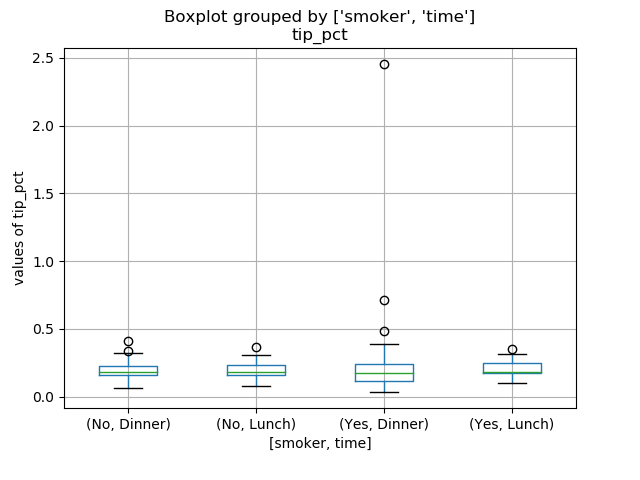DataFrame.boxplot()的用法具体参考：
https://pandas.pydata.org/pandas-docs/stable/generated/pandas.DataFrame.boxplot.html?highlight=dataframe%20boxplot#pandas.DataFrame.boxplot
方法2具体示例：
seaborn.cataplot()示例：
sns.catplot(x='tip_pct',y='day',hue='smoker',kind='box',
data=tips[tips.tip_pct < 0.5])
# hue表示分组的依据

fig.savefig('p4.png')    # 将绘制的图形保存为p4.png
上述代码绘制的图形为：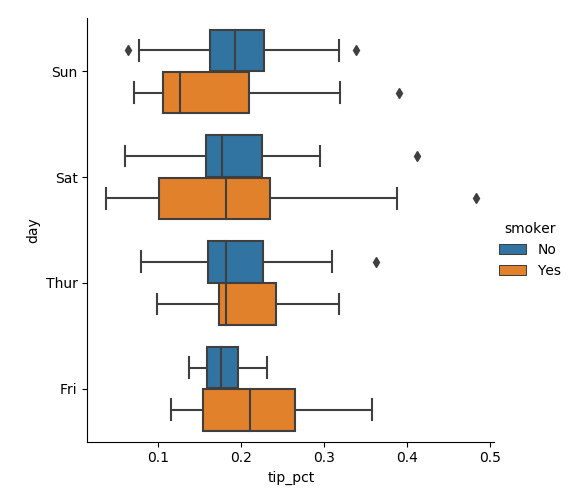seaborn.catplot()的用法具体参考：
http://seaborn.pydata.org/generated/seaborn.catplot.html?highlight=seaborn%20catplot#seaborn.catplot
seaborn.boxplot()示例：
fig,axes = plt.subplots()
sns.boxplot(x='day',y='tip_pct',hue='smoker',
data=tips[tips.tip_pct < 0.5],orient='v',ax=axes)
# orient参数表示箱形图的方向

axes.set_title('Boxplots grouped by smoker')
fig.savefig('p5.png')    # 将绘制的图形保存为p5.png
上述代码绘制的图形为：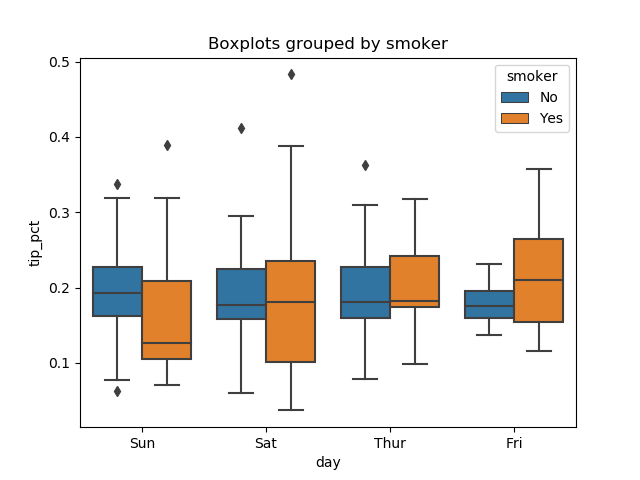seaborn.boxplot()的用法具体参考：
http://seaborn.pydata.org/generated/seaborn.boxplot.html#seaborn.boxplot
方法3具体示例：
axes.boxplot()示例：
fig,axes = plt.subplots()
axes.boxplot(x=tips.tip_pct,sym='rd',positions=)
# sym参数表示异常值的标记方式
# positions表示箱形图的位置标签

axes.set_xlabel('tip_pct')
fig.savefig('p6.png')    # 将绘制的图形保存为p6.png
上述代码绘制的图形为：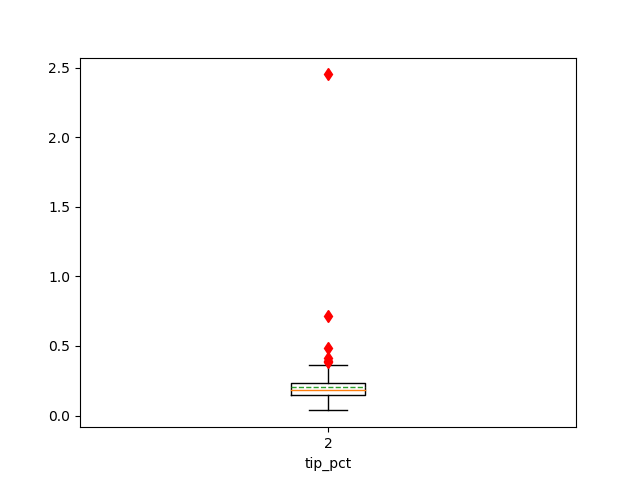axes.boxplot()的用法具体参考：
https://matplotlib.org/api/_as_gen/matplotlib.axes.Axes.boxplot.html?highlight=axes%20boxplot#matplotlib.axes.Axes.boxplot
其他参考资料：
《Python for Data Analysis》第二版
《商务与经济统计》第十三版
pandas、matplotlib、seaborn官方文档
PS：本文为博主原创文章，转载请注明出处。


展开全文数据分析
• 作者：小dull鸟来源：Python数据分析之禅Python绘制箱形图全解数据挖掘工作中的第一步就是异常值检测，异常值的存在会影响实验结果。箱形图主要是为了检测异常值，箱形图最大的优点就...作者：小dull鸟
来源：Python数据分析之禅

Python绘制箱形图全解
数据挖掘工作中的第一步就是异常值检测，异常值的存在会影响实验结果。箱形图主要是为了检测异常值，箱形图最大的优点就是不受异常值的影响，能够准确稳定地描绘出数据的离散分布情况，同时也利于数据的清洗。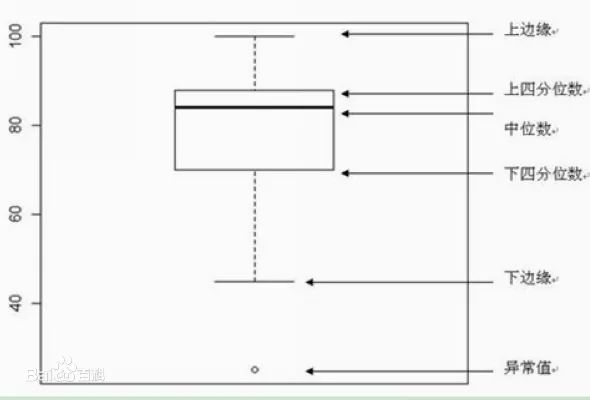举个例子:
有一组学生成绩数据：
[59, 60, 67, 70, 73, 76, 78, 85, 87, 90, 92, 540]

我们想看一下有没有手误输错的成绩，那么就可以用箱形图来判断
计算成绩数据的5种值：
1.下四分位数Q1
Qi所在位置=i（n+1）/4，其中i=1，2，3。n表示序列中包含的项数。
Q1所在的位置=（12+1）/4=3.25
Q1=0.75×第三项+0.25×第四项=0.75×67+0.25×70=67.75

2.中位数（第二个四分位数）Q2
Q1所在的位置=2（12+1）/4=6.5
Q1=0.5×第六项+0.5×第七项=0.5×76+0.5×78=115

3.上四分位数Q3
Q3所在的位置=3（12+1）/4=9.75
Q3=0.25×第九项+0.75×第十项=0.25×87+0.75×90=89.25

4.上限
上限是非异常范围内的最大值。
首先要知道什么是四分位距如何计算的？
四分位距IQR=Q3-Q1，那么上限=Q3+1.5IQR

5.下限
下限是非异常范围内的最小值
下限=Q1-1.5IQR

该学生成绩数据的箱形图可以这样画：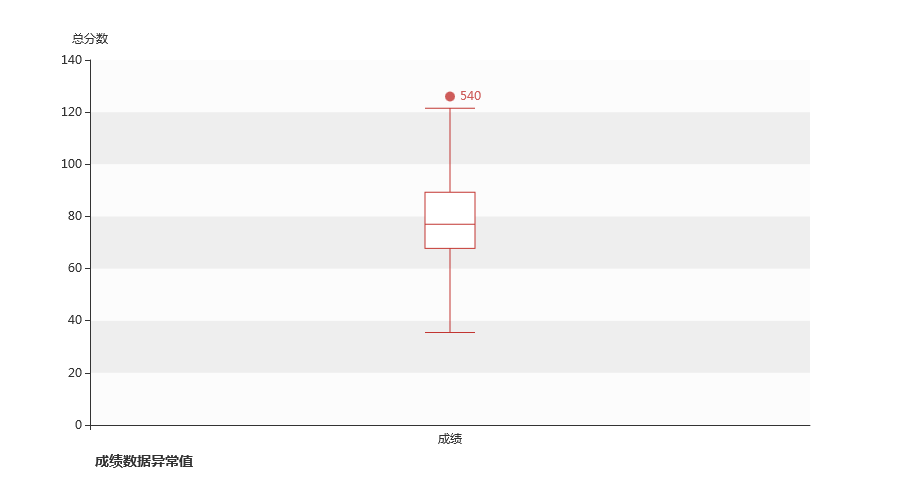发现有1个异常值，明显是手误打错的
上面这些过程可以直接用pyecharts实现，非常方便
一、基本箱形图
from pyecharts import options as opts
from pyecharts.charts import Boxplot

v1 = [
[850, 740, 900, 1070, 930, 850, 950, 980, 980, 880, 1000, 980],
[960, 940, 960, 940, 880, 800, 850, 880, 900, 840, 830, 790],
]
v2 = [
[890, 810, 810, 820, 800, 770, 760, 740, 750, 760, 910, 920],
[890, 840, 780, 810, 760, 810, 790, 810, 820, 850, 870, 870],
]
b=(
Boxplot()
.set_global_opts(title_opts=opts.TitleOpts(title="基本箱形图"))
)
b.render_notebook()




prepare_data可以对数据进行处理，得到上述的5种值
二、显示异常值的箱形图
经过研究发现，Boxplot的prepare_data模块不会判断并自动画出异常值，于是我对它进行了改进（源码在文末）
from pyecharts import options as opts
from pyecharts.charts import Boxplot,Scatter,Grid

y_data = [
[850,740,900,1070,930,850,950,980,80,880,1000,980,930,650,760,810,1000,1000,960,960,],
[960,940,960,940,880,800,850,880,900,840,830,790,810,880,880,830,800,790,760,800,],
[880,880,880,860,720,720,620,860,970,950,880,910,850,870,840,840,850,840,840,840,],
[890,810,810,820,800,770,760,740,750,760,910,920,890,860,880,720,840,850,850,780,],
[890,840,780,810,760,810,790,810,820,850,870,870,810,740,810,940,950,800,810,870,],
]
box_plot = Boxplot()
data1, scatter_datas=box_plot.prepare_data(y_data)  #对prepare_data模块进行改进，返回两个列表
box_plot = (
.set_global_opts(
xaxis_opts=opts.AxisOpts(
type_="category",
boundary_gap=True,
splitarea_opts=opts.SplitAreaOpts(is_show=False),
splitline_opts=opts.SplitLineOpts(is_show=False),
),
yaxis_opts=opts.AxisOpts(
type_="value",
name="总分数",
splitarea_opts=opts.SplitAreaOpts(
is_show=True, areastyle_opts=opts.AreaStyleOpts(opacity=1)
),
),
)
.set_series_opts(tooltip_opts=opts.TooltipOpts(formatter="{b}: {c}"))
)

scatter = (
Scatter()
.set_global_opts(
title_opts=opts.TitleOpts(
pos_left="10%",
pos_top="90%",
title="成绩数据异常值",
title_textstyle_opts=opts.TextStyleOpts(
border_color="#999", border_width=1, font_size=14
),
),
yaxis_opts=opts.AxisOpts(
axislabel_opts=opts.LabelOpts(is_show=False),
axistick_opts=opts.AxisTickOpts(is_show=False),
),
)
)

grid = (
Grid()
box_plot,
grid_opts=opts.GridOpts(pos_left="10%", pos_right="10%", pos_bottom="15%"),
)
scatter,
grid_opts=opts.GridOpts(pos_left="10%", pos_right="10%", pos_bottom="15%"),
)
)

grid.render_notebook()




对prepare_data模块进行改进，返回两个列表,第一个是和之前的5个值一样，第二个是异常值组成的列表。
-完-
完整代码请扫码关注公众号并回复“20200921”获取展开全文数据挖掘 数据分析 css html 数据可视化
• 如下灰色框里的就是箱形图（英文：Box plot）：又称为盒须图、盒式图、盒状图或箱线图，是一种用作显示一组数据分散情况资料的统计图。因型状如箱子而得名。 箱形图最大的优点就是不受异常值的影响，可以以一种相对...数据挖掘
• 箱形图系列箱形图(box plot)也称箱须图(box-whisker plot)、箱线图、盒图显示一组数据的最大值、最小值、中位数、及上下四分位数；用来反映一组或多组连续型定量数据分布的中心位置和散步范围；从盒子两端延伸出来的...
• column:需要绘制箱形图的列名，默认为展示全部数字型列的箱型图 fontsize：横坐标字体大小 rot:横坐标字体的旋转角度 grid:背景网格，默认为展示 figsize ：输出箱型图的大小，格式为（长，宽） by:分组绘图的列名，...
• python绘制箱型十分简单，而且有很多种方法可以实现，可以看看这篇文章，多种python箱型绘制方法。但是奇怪的是网上竟然都搜不到分组绘制箱型的方法。所谓分组绘制箱型就是首先对数据进行一个groupby操作，...seaborn
• ## Python绘制箱形图

千次阅读 2019-06-13 17:51:46
import numpy as np import matplotlib.pyplot as plt age = [23,23,27,27,39,41,47,49,50,52,54,54,56,57,58,58,61] fat = [9.5,26.5,7.8,17.8,31.4,25.9,27.4,27.2,31.2,34.6,42.5,28.8,33.4,30.2,34.1,23.9,35.7...
• 箱形图的知识点箱型图优点箱型图缺点箱形图的五要素 箱型图优点 箱型图常用于检测并展现出数据中的异常值，利用箱型图可以判断数据的偏态和尾重。（即观测数据的整体集中分布情况，当数据分布较为集中的时候，箱型...数据可视化 数据分析
• 箱形图：从字面上理解就是箱子的图，如下图： 箱形图有5个参数： 下边缘（Q1），表示最小值； 下四分位数（Q2），又称“第一四分位数”，等于该样本中所有数值由小到大排列后第25%的数字； 中位数（Q3），又称...统计学 异常检测
• 最近在学习使用Python画图，想用subplot画两幅箱型，分别用来表示三组数据在空间滤波前后WRMS值的变化情况。关于Pycharm的安装和配置可以自行百度，直接贴上代码，给出效果。代码中给出标注以便日后查询。 ...boxplot
• 箱形图（英文：Box plot），又称为盒须图、盒式图、盒状图或箱线图，是一种用作显示一组数据分散情况资料的统计图。因型状如箱子而得名。在各种领域也经常被使用，常见于品质管理，快速识别异常值。 箱形图最大的...数据分析
• 主要介绍了Python箱型绘制与特征值获取过程解析,文中通过示例代码介绍的非常详细，对大家的学习或者工作具有一定的参考学习价值,需要的朋友可以参考下
• 同时画出多个箱形图 import numpy as np import matplotlib.pyplot as plt np.random.seed(100) data = np.random.normal(size=(1000,4),loc=0,scale=1) labels = ['A','B','C','D'] plt.boxplot(data,labels =...
• 0.篇首本篇文章继续在 Iris 的基础上做数据可视化处理。...本篇文章将从统计学常用的几个概念出发，引出今天的主角 —— 箱形图1.箱形图首先，统计学中，针对一个数据样本，我们往往会用平均数 mean...iris数据
• python_循环为每个特征做箱型 # python_循环为每个特征做箱型 data_temp = data_sample def box(dataset,columns): fig_num = len(columns) sub_figure = [fig_num//5+1,5] # figsize : 2-tuple of floats, ...
• 其中，大家都可能非常清楚条形图、直方图、散点图和曲线图的用处，但是箱形图呢？（或者称为盒须图）。他的意义在哪里呢？在python中又是如何实现的呢？于是我今天翻开了贾俊平老师的那本《统计学》琢磨了一下午，又...数据分析
• 箱型比较复杂，这里只做简要介绍。 声明： 需要读者了解Python列表 最好了解Python的pandas库 教程借助于matplotlib绘图库
• 今天小编就为大家分享一篇解决python中用matplotlib画多幅时出现图形部分重叠的问题，具有很好的参考价值，希望对大家有所帮助。一起跟随小编过来看看吧
• 2. 小提琴（箱线图和密度的结合） sns.violinplot() L = [ 9 , 3 , 1 , 5 , 3 ] sns . violinplot ( y = L ) # x = L 可以将小提琴横过来 sns . violinplot ( x = 'day' , y = '...seaborn
• 箱形图 箱形图（英文：Box plot），又称为盒须图、盒式图、盒状图或箱线图，是一种用作显示一组数据分散情况资料的统计图。因型状如箱子而得名。在各种领域也经常被使用，常见于品质管理，快速识别异常值。 箱形图...深度学习
• 箱形图 概念 用处 箱形图系列模板 第一个箱形图 复杂一点的图例 每文一语 箱形图 概念 后面的图形都是一些专业的统计图形，当然也会是我们可视化的对象。 箱形图（Box-plot）又称为盒须图、盒式图或箱线图...数据分析 可视化 pyecharts
• 举例说明，以下是箱形图的具体例子： 这组数据显示出： 最小值(minimum)=5 下四分位数(Q1)=7 中位数(Med--也就是Q2)=8.5 上四分位数(Q3)=9 最大值(maximum)=10 平均值=8 四分位间距=Q3-Q1=2 (即ΔQ) 最大值区间： ...
• 方法一： 采用pandas里的plot.box import numpy as np import pandas as pd import matplotlib.pyplot as plt data = { 'label1':data1, 'label2':data2, 'label3':data3 ...plt.grid(linestyle="--", alpha开发语言 后端 boxplot pandas
• 利用python进行数据分析，matplotlib数据可视化，绘制箱形图、散点图和直方图。matplotlib 散点图 直方图...

# 箱型图pythonpython 订阅